## 7.6Alternative wall functions

The standard wall function described in Sec. 7.5 uses a function for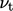that is discontinuous at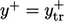, switching to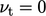for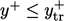. A continuous wall function is available which evaluatesas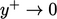from a single equation describing the universal character of the velocity proﬁle at high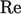,9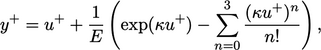(7.22)
where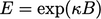. The equation combines Eq. (7.11 ) and Eq. (7.13 ), “disabling” Eq. (7.13 ) at low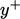by subtracting low order terms from a polynomial expansion of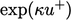.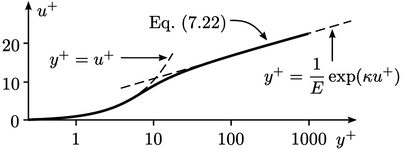The wall function is applied by solving Eq. (7.22 ) for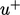from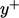calculated from Eq. (7.9) using the near-wall cell centre height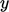. The friction velocity is calculated by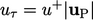, where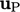is the near-wall cell velocity. Finally,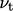on the wall patch is calculated from a numerical interpretation of Eq. (7.20 ),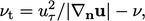(7.23)
where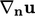is the surface-normal velocity gradient. An iterative method is required to invert Eq. (7.22) and to accommodate other nonlinearities, e.g.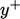is itself a function of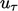.

### Rough wall function

The standard wall function in Sec. 7.5 is applicable to smooth walls so does not account for surface roughness. Roughness is signiﬁcant when the roughness “scale”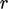10 becomes larger than the thickness of the viscous sub-layer.

At higher surface roughness, turbulent eddies are generated near the wall at a scale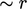, rather than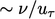. The viscous eﬀects become negligible, causing the non-dimensionalised distance to become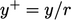in the log law Eq. (7.13 ). To reﬂect this, Eq. (7.13 ) is modiﬁed to a form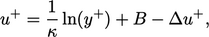(7.24)
where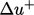a roughness function, dependent on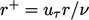. An intuitive model foris11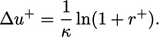(7.25)
This rough wall function Eq. (7.24 ) reduces to Eq. (7.13 ) using the conventional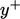deﬁnition of Eq. (7.9 ) at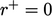. As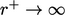, it reduces to Eq. (7.13 ) using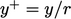.

It is open to interpretation how to determine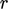from roughness measurements of a surface. The parameter is sometimes split into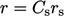, where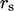is a measured sand grain roughness height and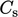is a coeﬃcient that depends on the shape, consistency and packing of the roughness elements. Using that approach, values of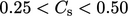often yield a good match between Eq. (7.24 ) and measured data.

9Brian Spalding, A single formula for the law of the wall, 1961.
10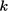’ is often used to denote roughness, but we use ‘’ to avoid confusion with turbulent kinetic energy.
11Cyril Colebrook, Turbulent ﬂow in pipes, with particular reference to the transitional region between smooth and rough wall laws, 1939.

Notes on CFD: General Principles - 7.6 Alternative wall functions